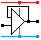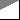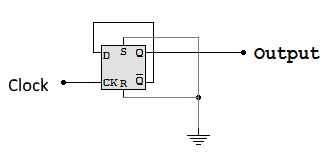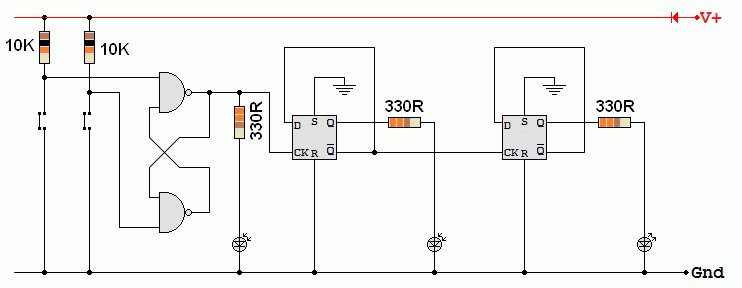Home

rOm
Quest
Glossary

Random
Page
Search
Site
Lush
Sim
Class
Subject
Images

Help
FAQ
Sign
Up
Log
In
 GCSE     Sequential Logic     BCD Counting     D Type Flip Flop     D Type Latch     Decade Counter     Sequencer     >Up Counter<

# Sequential Logic Up Counter

Site for Eduqas/WJEC - Go to the AQA site.

GCSE    Sequential LogicQuestions 0 to 32   -->  View All

On the rising edge of the clock pulse, the input (D) is copied to the output (Q).
Since Q is connected to D, the output is inverted on the rising edge.

A ripple counter is an asynchronous counter where only the first flip-flop is clocked by an external pulse.
All subsequent flip-flops are clocked by the output of the preceding flip-flop.
The counter output might be momentarily wrong until the clock pulse has reached every the flip-flop.
This takes about 20 nanoseconds per flip-flop.

## A One Bit Counter using a D Type Flip Flop

In decimal this counts 0 1 0 1 0 1 and in binary, it's the same.• The output of this counter has half the frequency of the input so this is also a frequency divider.
• The output has an exact 50:50 or one to one Mark Space Ratio, whatever shape the clock signal has.

View counter in the Lushprojects Simulator.

## A Two Bit Counter using Two D Type Flip Flops

In decimal, this counter counts 0 1 2 3 0 1 2 3 0 1 2 3 and in binary, 00 01 10 11 00 01 10 11 00 01 10 11 00 01 10 11 .View counter in the Lushprojects Simulator.

## Frequency Divider

On the rising edge of the clock pulse, D is copied to Q.
Since NOT Q is connected to D, the data is inverted on each rising edge.
This has the effect of dividing the frequency by two.

• The D Type Flip Flop is used in Binary Counters.
• Here is the circuit for a one bit counter.
• This can also be used as a frequency divider. It divides the frequency by two.
• The UP in Up Counter is because the counter counts normally with increasing numbers like 0, 1, 2, 3 etc.
• The output of this circuit is high for 50% of the time and low for 50% of the time.
• This is a 1:1 mark space ratio.
• This is true whatever the mark space ratio of the clock pulses.## Switch Debounce and a One Bit Counter - Build This

The two NAND gates are connected as a Bistable Flip Flop. This is used to debounce the switch pulses. The 4013 D Type Flip Flop is wired as a one bit binary counter. Each time the Bistable LED comes on (the rising edge), the counter LED changes state. This counter counts 0, 1, 0, 1, Etc.This is a slightly odd circuit because two different families of logic chips are being used.

• The 74xx series chips run on 4.75 to 5.25 Volts and consume more power.
• The 40xx series chips are less fussy about the power supply voltage and they consume less power.
• The 7400 could be replaced with a 4011 chip but the pinout is not the same so some re-wiring would also be needed.## A Two Bit Counter - Build this if you have time.• This circuit adds a second stage to the counter. The two stages now count 0, 1, 2, 3, 0, 1, 2, 3, Etc.
• Every time a new stage is added, the count upper limit doubles. 1, 3, 7, 15, 31, 63 Etc.
• A seven stage counter counts up to 2N - 1    =     27 - 1    =    127

Subject Name     Level     Topic Name     Question Heading     First Name Last Name Class ID     User ID

 CloseEscapeX Q: qNum of last_q     Q ID: Question ID         Score: num correct/num attempts         Date Done

Question Text

image url

Help Text
Debug

• You can attempt a question as many times as you like.
• If you are logged in, your first attempt, each day, is logged.
• To improve your scores, come back on future days, log in and re-do the questions that caused you problems.
• In the grade book, you can delete your answers for a topic before re-doing the questions. Avoid deleting unless you intend re-doing the questions very soon.